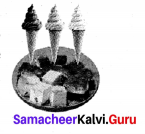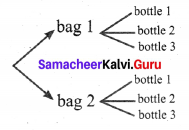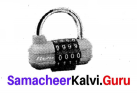# Samacheer Kalvi 8th Maths Solutions Term 1 Chapter 5 Information Processing Ex 5.1

Students can Download Maths Chapter 5 Information Processing Ex 5.1 Questions and Answers, Notes Pdf, Samacheer Kalvi 8th Maths Book Solutions Guide Pdf helps you to revise the complete Tamilnadu State Board New Syllabus and score more marks in your examinations.## Tamilnadu Samacheer Kalvi 8th Maths Solutions Term 1 Chapter 5 Information Processing Ex 5.1

Question 1.
How many outcomes can you get when you toss three coins once?
(A) 6
(B) 8
(C) 3
(D) 2
Solution:
(B) 8

Question 2.
In how many ways can you answer 3 multiple choice questions, with the choices A, B, C and D ?
(1) 4
(2) 3
(3) 12
(4) 64
Solution:
(4) 64

Question 3.
How many 2 digit numbers contain the number 7 ?
(A) 10
(B) 18
(C) 19
(D) 20
Solution:
(B) 18Question 1.
You are going to have an ice cream or a cake. There are three flavours (chocolate, strawberry , vanilla) in ice creams, and two flavours (orange or red velvet) in the cake. In how many possible ways can you choose an ice cream or the cake?Solution:
We are going to have either a ice cream or a cake.
Ice cream can be selected from 3 flavors and cake from two flavors. Both the events cannot occur simultaneously selecting ice cream and cake.
∴ Number of possible ways = 3 + 2
= 5 ways

Question 2.
In how many ways, can the teacher choose 3 students, in all one each from 10 students in VI std, 15 students in VII std and 20 students in VIII std to go to an excursion?
Solution:
The teacher is going to selected one student from class VI out of 10 students in 10 ways from
class VII out of 15 students in 15 ways and from class VIII out of 20 students in 20 ways.
∴ Number of ways 3 students can be selected
= 10 + 15 + 20 = 45 ways

Question 3.
If you have 2 school bags and 3 water bottles then, in how many different ways can you carry a school bag and a water bottle, while going to school?
Solution:
We can select one school bag from 2 and one bottle from 3 as follows.∴ A bag and a water bottle can be selected in 2 × 3 = 6 ways.Question 4.
Roll numbers are created with a letter followed by 3 digits in it. From the letter A, B, C, D, E and any 3 digits from 0 to 9. Then, in how many possible ways can the roll numbers be generated?
Solution:
We have a letter followed by 3 digits in the roll number.
The letter is selected from A, B, C, D, E.
For these 5 letters we have to select a 3 digit number using the digits 0 to 9.
Once place can be formed using any one of the 10 number 0 to 9 in 10 ways.
Tens place can be formed in 10 ways.
∴ A two digit number can be formed in 10 × 10 = 100 ways.
Thousands place can be formed in 10 ways
∴ A 3 digit number can be formed in 10 × 10 × 10 = 1000 ways.
∴ 5 letters can be attached in 5 × 1000 = 5000 ways.
∴ The roll number can be formed in 5000 ways.

Question 5.
A safety locker in a jewel shop requires a 4 digit unique code. The code has the digits from 0 to 9. How many unique codes are possible ?Solution:
The unique code has 4 digits.
Each digit is formed using any of the 10 numbers from 0 to 9.
∴ Single digit number can be formed in 10 ways.
A double digit number can be formed in 10 × 10 ways.
A three digit number can be formed in 10 × 10 × 10 ways.
A four digit number can be formed in 10 × 10 × 10 × 10 ways. = 10,000 ways